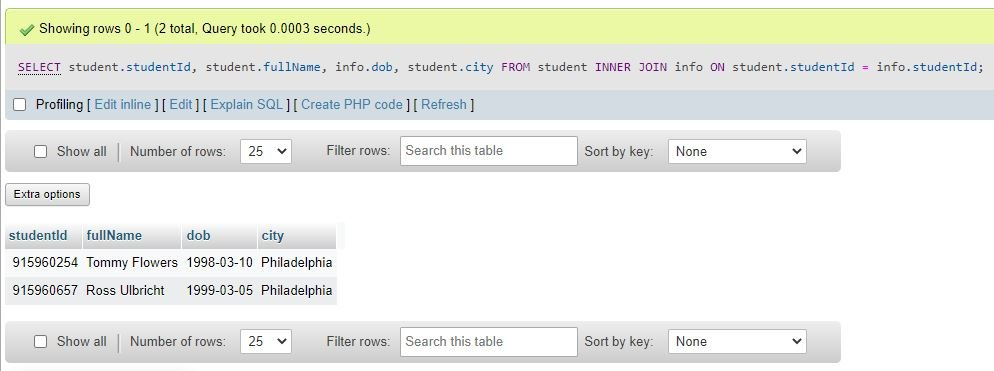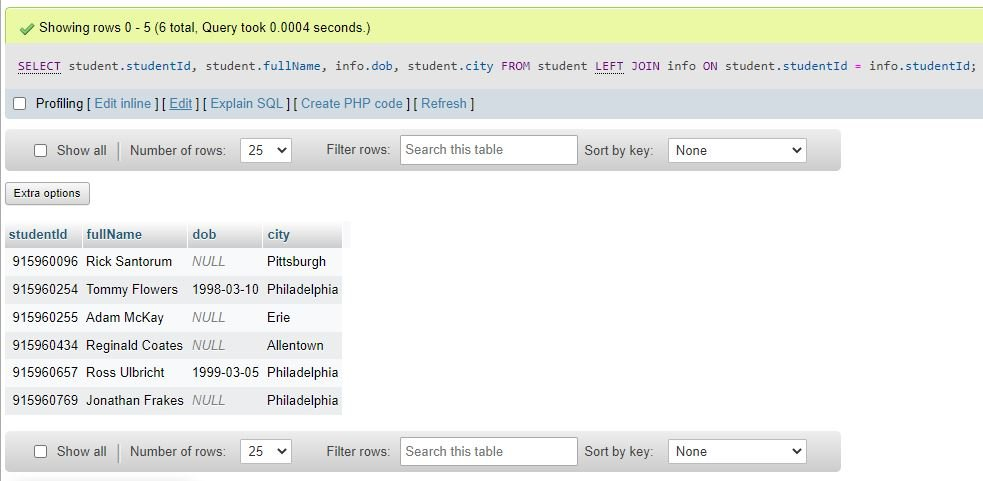# Combine Two Tables in SQL | SQL JOIN Table

Sometimes we need to join or combine two tables based on the column that is common between both tables. To achieve this task, SQL provides the JOIN clause.

Before we proceed to describe about the joining of two tables using the SQL JOIN clause, let me create two tables for you to take the examples, so that you can easily understand the topic ☺.

Now I just created the two tables, namely "student" and "info." Here is a snapshot of the "student" table for your understanding.And the snapshot of the second table named "info" isNow let me write a SQL query as an example to show you how to join two tables using the JOIN clause.

```SELECT student.studentId, student.fullName, info.dob, student.city
FROM student
JOIN info ON student.studentId = info.studentId;```

The above SQL query displays the columns "studentId,"  "fullName,"  and "city" from the table "student" and "dob" from the table "info." Concentrate on the order of columns that I have listed in the above SQL query. Since the column "studentId" is written first, it will be displayed first, whereas the column "city" is written last, therefore this column will be displayed last.

Now the following SQL code snippet from the above SQL example:

`JOIN info ON student.studentId = info.studentId;`

is used to display all the records from both tables with a matching "studentId" column value. Since we have the two studentIds (915960254 and 915960657, respectively) available in both tables, Therefore, we will get specified data from these two rows.

Here is the output that will be produced after executing the above SQL query on joining two tables:We can use other keywords along with JOIN, such as LEFT, RIGHT, or FULL, to modify the joining criteria. For example, use LEFT JOIN to display all records from the left and matched records from the right table; use RIGHT JOIN to display all records from the right and matched records from the left; or use FULL JOIN to display all records if a match is found either from the left or from the right table. For example: the following SQL query:

```SELECT student.studentId, student.fullName, info.dob, student.city
FROM student
LEFT JOIN info ON student.studentId = info.studentId;```

will produce the following output:SQL Online Test

« Previous Topic Next Topic »